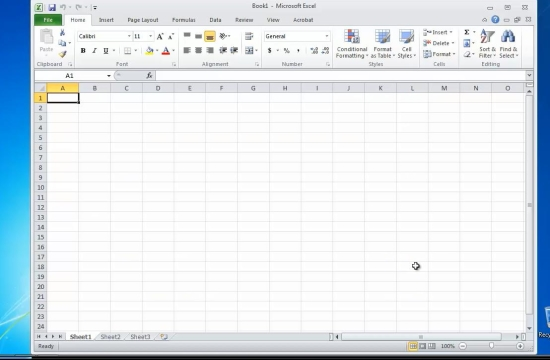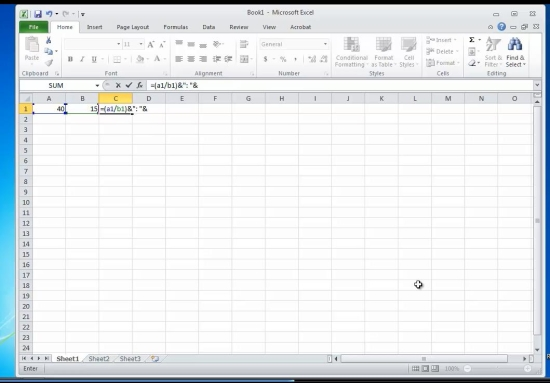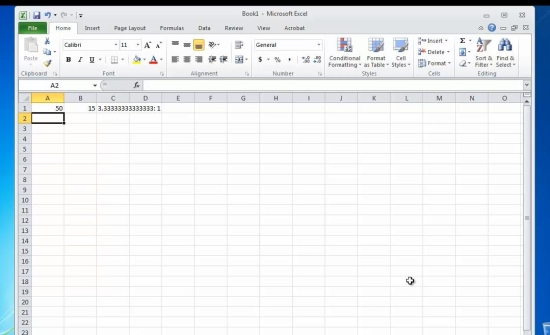## How to Calculate the Ratio in Excel

In this tutorial you will learn how to calculate ratio in Excel

A comparative function, the ratio value is the relationship between two or more numbers and is shown by the signs of : or /
Mathematically, it can be transformed into a comparison after the division of the numbers by the smallest of them.

Prerequisites: Microsoft Excel

Step 1. Launch Excel and start a new file from File- New.Step 2. Type the numbers you want to calculate the ration for in two adjacent cells.Step 3. Type in the formula:

=INT (A/B)&” : ”&(B/B), where

A and B are the cell coordinates of your two numbers.Result: Congratulations, you have learnt how to calculate the ratio in Excel.